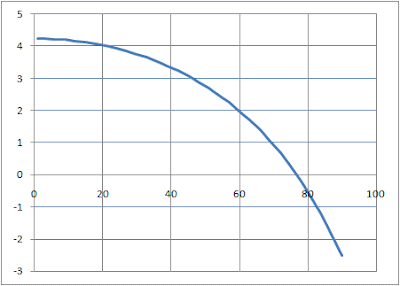## Monday, November 19, 2007

### Soonest-landing trajectory

In my rocket game, it is difficult to know when to begin a braking burn in order to land at a given target. I've been working on the math for a trajectory that lands at the nearest possible point. (To clarify: I want the nearest point that doesn't involve backtracking or waiting for the next orbit. Given unlimited fuel and time you can land anywhere.)

To start with I'm making some simplifying assumptions. These boil down to assuming you're close to the ground. Gravity is constant; it doesn't vary with position. The ground is a flat plane at y=0, and the gravity vector is pointed in the -y direction. With the constant-gravity assumption, the rocket's trajectory becomes a parabola and can be solved easily.

If the rocket starts out high enough, the trajectory is simple. The rocket is pointed completely sideways and fired until the horizontal velocity is zero. After that it is turned upright, falls a bit if necessary, and then fires vertically to brake to a soft landing.

A sample rocket trajectory of this type is plotted below. For this graph, the rocket's acceleration is 2 and its initial velocity is (4, 0). Gravity acceleration is -1. The graphs end when horizontal velocity reaches zero; they do not show the vertical braking component (if any).Sometimes, though, canceling all horizontal velocity before addressing vertical velocity doesn't work because the rocket falls too far and hits the ground before it's done, or else there isn't enough altitude, given its vertical velocity, to complete the vertical braking. In these cases the rocket has to be tilted upward somewhat, diverting some acceleration toward staying above ground. We want to divert the minimum necessary, so as to extend the braking distance as little as possible.

In the example below, the rocket acceleration is still 2, but the initial velocity is increased to (6, 0). As a result, the rocket has to be tilted at an angle of 76.86 degrees from vertical in order to have space for the vertical descent:Finally, sometimes the initial velocity is such that the rocket has to actually gain altitude in order to make a soft landing. Here, I've reduced the rocket's acceleration to 1.25, set the initial velocity to (6, -0.5), and put the initial rocket altitude at 1. The rocket angle is 25.84 degrees from vertical:I've let the rocket graze the ground in the trajectory above. In reality you'd pick a safe minimum altitude and adjust the angle to keep it above that.

Thus, the rocket's trajectory consists of one or two pieces. If the horizontal velocity is nonzero, the rocket will execute a burn while tilted to the side by some amount. Once the horizontal velocity is zero, if there is remaining elevation, there will be a vertical burn to cancel that. (There may be a coasting period in between the two burns.) For simplicity I'm assuming that the rocket can change heading instantly. Time for turning could be added to the problem without too much trouble.

I've covered the coast-and-vertical-braking phase in a previous entry. The remaining problem is to find the angle for the first burn. The general approach is as follows:

For a given angle of the rocket, we can compute when it will cancel its horizontal velocity (divide horizontal velocity by horizontal acceleration). We plug this time into the equations for vertical motion to find out the rocket's elevation and vertical velocity at that time. This elevation and velocity can then be plugged into the equation for the altitude at which to begin vertical braking, developed in the above-mentioned blog entry. If the braking altitude is at or below us, we're good. If it's above us, we can't do it, and this angle is not usable.

Since we're comparing two altitudes, the natural thing to do is to make an equation which says that their difference equals zero. By solving this we get the minimum angle which will result in a successful landing. The minimum angle equals minimum distance traveled since we put as much thrust as possible toward horizontal braking.

Unfortunately since the variable we're solving for is encased in sines and cosines I don't know if there's an analytical way to solve for it. However, it should be easy to search for the value. Here's a sample plot illustrating the type of curve it is:The curve above corresponds to the second case given earlier. It crosses the x axis at around 76.86 degrees.

The only remaining wrinkle (that I know of) is presented in the third case above. We can't ever let the rocket's elevation dip below zero. We need to determine when its vertical velocity reaches zero (this is a possible point of minimum altitude). If this time is earlier than the time for zero horizontal velocity (and greater than zero), then we need to check the rocket's elevation at that time. If it's less than zero (or whatever safe altitude we choose), then we need to search for a rocket angle closer to vertical that keeps the rocket above-ground.

I've been working this out in Excel; the next thing to do is to implement it in-game. There are a variety of ways it could be used: I could plot the earliest-landing point on the ground, or plot the trajectory to it, or have an attitude indicator showing which direction to burn. Once it's in I'll see how valid the flat-earth assumptions are. They do become more correct as the rocket approaches the ground so they may not be a problem.

Another thing this could be useful for is the beginning of a rocket AI. Computer-controlled rockets will probably have slightly different trajectory needs. They'll be wanting to land at a specific spot, rather than at the earliest possible spot. It shouldn't be too different, though.

#### 1 comment:jon w said...

hey man, nice blog :)